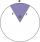# Cosine - math problems

#### Number of problems found: 149

• ConeCalculate volume and surface area of ​​the cone with a diameter of the base d=15 cm and side of the cone with the base has angle 52°.
• House roofThe roof of the house has the shape of a regular quadrangular pyramid with a base edge 17 m. How many m2 is needed to cover roof if roof pitch is 57° and we calculate 11% of waste, connections and overlapping of area roof?
• Two boatsTwo boats are located from a height of 150m above the surface of the lake at depth angles of 57° and 39°. Find the distance of both boats if the sighting device and both ships are in a plane perpendicular to the surface of the lake.
• Two forcesThe two forces F1 = 580N and F2 = 630N, have an angle of 59 degrees. Calculate their resultant force, F.
• Bearing - navigationA ship travels 84 km on a bearing of 17° and then travels on a bearing of 107° for 135 km. Find the distance of the end of the trip from the starting point, to the nearest kilometer.
• A trapezoidA trapezoid with a base length of a = 36.6 cm, with angles α = 60°, β = 48° and the height of the trapezoid is 20 cm. Calculate the lengths of the other sides of the trapezoid.
• Resultant forceCalculate mathematically and graphically the resultant of three forces with a common center if: F1 = 50 kN α1 = 30° F2 = 40 kN α2 = 45° F3 = 40 kN α3 = 25°
• MastMast has 13 m long shadow on a slope rising from the mast foot in the direction of the shadow angle at angle 13.3°. Determine the height of the mast, if the sun above the horizon is at angle 45°12'.
• Two forcesTwo forces with magnitudes of 25 and 30 pounds act on an object at 10° and 100° angles. Find the direction and magnitude of the resultant force. Round to two decimal places in all intermediate steps and your final answer.
• Circular sectorI have a circular sector with a length 15 cm with an unknown central angle. It is inscribed by a circle with radius 5 cm. What is the central angle alpha in the circular sector?
• Rotary coneThe volume of the rotation of the cone is 472 cm3 and angle between the side of the cone and base angle is 70°. Calculate lateral surface area of this cone.
• Cylinder horizontallyThe cylinder with a diameter of 3 m and a height/length of 15 m is laid horizontally. Water is poured into it, reaching a height of 60 cm below the axis of the cylinder. How many hectoliters of water is in the cylinder?
• Triangle KLBIt is given equilateral triangle ABC. From point L which is the midpoint of the side BC of the triangle it is drwn perpendicular to the side AB. Intersection of perpendicular and the side AB is point K. How many % of the area of the triangle ABC is area o
• ForcesIn point O acts three orthogonal forces: F1 = 20 N, F2 = 7 N, and F3 = 19 N. Determine the resultant of F and the angles between F and forces F1, F2, and F3.
• The roofThe roof of the tower has the shape of a regular quadrangular pyramid, the base edge of which is 11 m long and the side wall of the animal with the base an angle of 57°. Calculate how much roofing we need to cover the entire roof, if we count on 15% waste
• Elevation anglesFrom the endpoints of the base 240 m long and inclined at an angle of 18° 15 ', the top of the mountain can be seen at elevation angles of 43° and 51°. How high is the mountain?
• Space diagonal anglesCalculate the angle between the body diagonal and the side edge c of the block with dimensions: a = 28cm, b = 45cm and c = 73cm. Then, find the angle between the body diagonal and the plane of the base ABCD.
• Inner anglesThe inner angles of the triangle are 30°, 45° and 105° and its longest side is 10 cm. Calculate the length of the shortest side, write the result in cm up to two decimal places.
• Pentagonal prismThe regular pentagonal prism is 10 cm high. The radius of the circle of the described base is 8 cm. Calculate the volume and surface area of the prism.
• SailboatThe 20 m long sailboat has an 8 m high mast in the middle of the deck. The top of the mast is fixed to the bow and stern with a steel cable. Determine how much cable is needed to secure the mast and what angle the cable will make with the ship's deck.

Do you have an interesting mathematical word problem that you can't solve it? Submit a math problem, and we can try to solve it.

We will send a solution to your e-mail address. Solved examples are also published here. Please enter the e-mail correctly and check whether you don't have a full mailbox.

Please do not submit problems from current active competitions such as Mathematical Olympiad, correspondence seminars etc...